Shape Parameters - Basic Properties edit page

In this section we discuss basic geometric properties of grains. Due to the huge amount of shape parameters we split them into different categories: basic properties (this page), properties based on fitted ellipses, convex hull bases properties, projection bases properties. The table below summarizes the shape parameters discussed on this page.

 grainSize number of pixels per grain grain area in µm² number of boundary segments perimeter in µm number of inner boundaries length of inner boundaries in µm diameter in µm caliper or Feret diameter perimeter of a circle with the same area radius of a circle with the same area perimeter / equivalent perimeter is it a boundary grain irregularity of grain boundary has inclusions is an inclusions number neighboring grains list of triple points list of boundary segments subgrain boundaries x, y coordinates of the vertices x,y coordinates of the barycenter

We start our discussion by reconstructing the grain structure from a sample EBSD data set.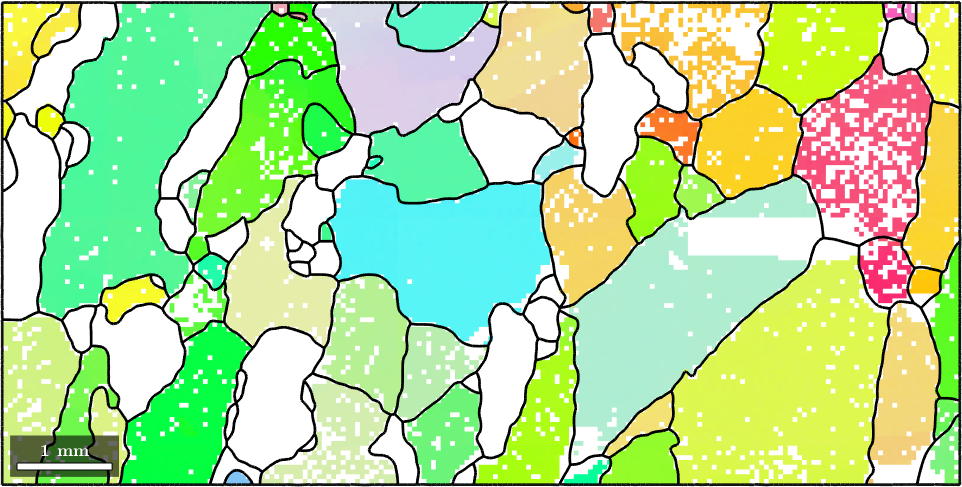## Grain size vs. grain area and boundary size vs. perimeter

The most basic properties are grainSize and grain area. Those can be computed by

Hereby grainSize referes to the number of pixels that belong to a certain grain while area represents the actual area measured in (µm)^2. We may analyze the distribution of grains by grain area using a histogram.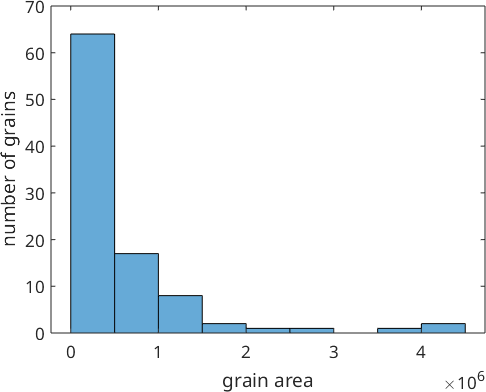Note the large amount of very small grains. A more realistic histogram we obtain if we do not plot the number of grains at the y-axis but its total area. This can be achieved with the command histogram(grains) or hist(grains)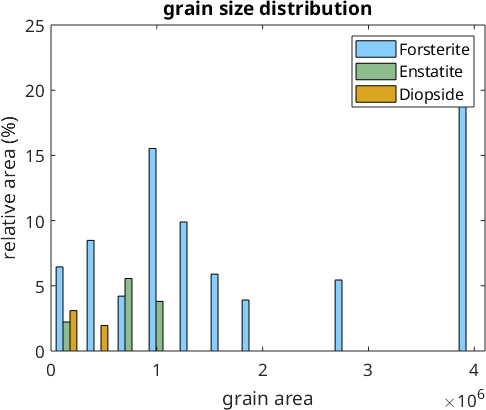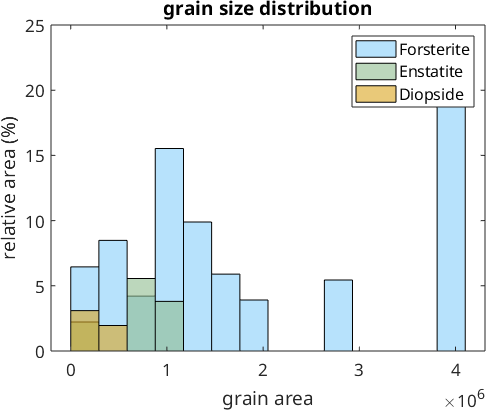Simarly as grainSize and area, the one-dimensional meassures boundarySize and perimeter give the length of the grain boundary as number of segments and in µm, respectively.

We may compute these quantities also explicitely from the grain boundary segents. In the following code the first command returns simply the number of boundary segments while the second one gives the total length of the boundary by summing up the length of each individual boundary segment

Another, one dimensional measure is the diameter which refers to the longest distance between any two boundary points and is given im µm as well

The diameter is a special case of the caliper or Feret diameter of a grain that is explained in detail in the section Projection based parameters.

In contrast the equivalent radius is the radius of a circle with the same area as the grain. Naturally, the equivalent radius is always smaller than the actual radius of the grains. Similarly, the equivalent perimeter is defined as the perimeter of the circle the same area and is always smaller then the actual perimeter.

As a consequence, the ratio between between actual grain perimeter and the equivalent perimeter, the so called shape factor, is always larger then 1. The shapeFactor amd measures how different a certain shape is from a circle.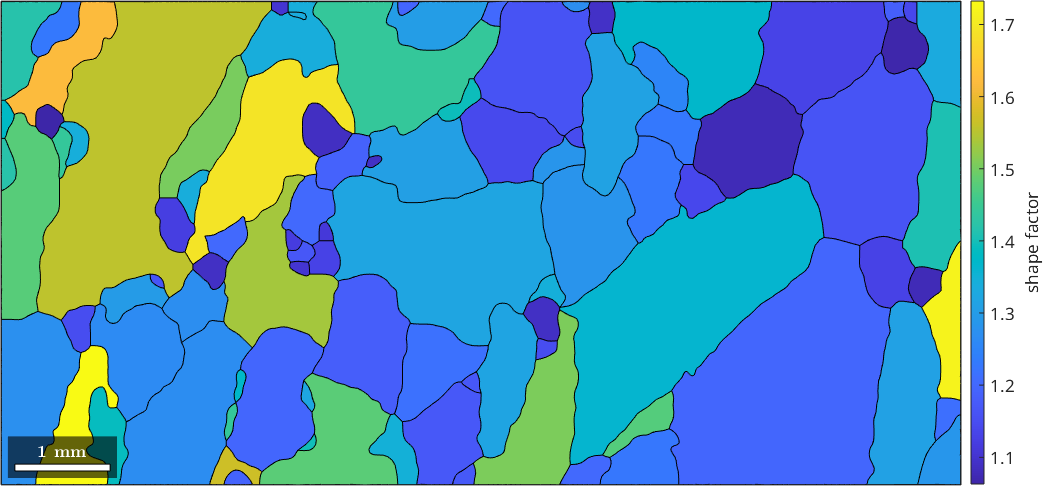A second measure for the discrepancy between the actual shape and a circle is the relative difference between the perimeter and the equivalentPerimeter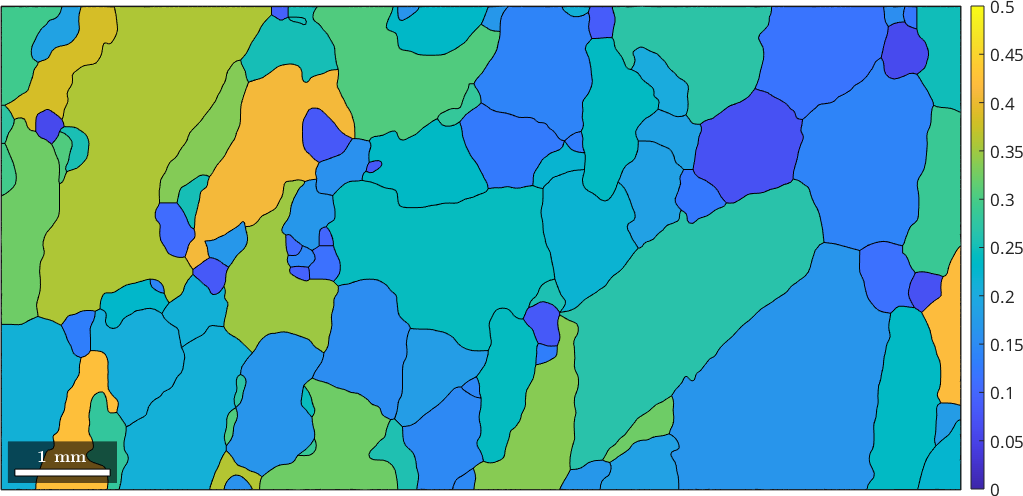In this plot round shapes will have values close to zero while concave shapes will get values up to $$0.5$$.

A third measure is the paris which stands for Percentile Average Relative Indented Surface and gives the relative difference between the actual perimeter and the perimeter of the convex hull. It is explained in more detail in the section convex hull parameters.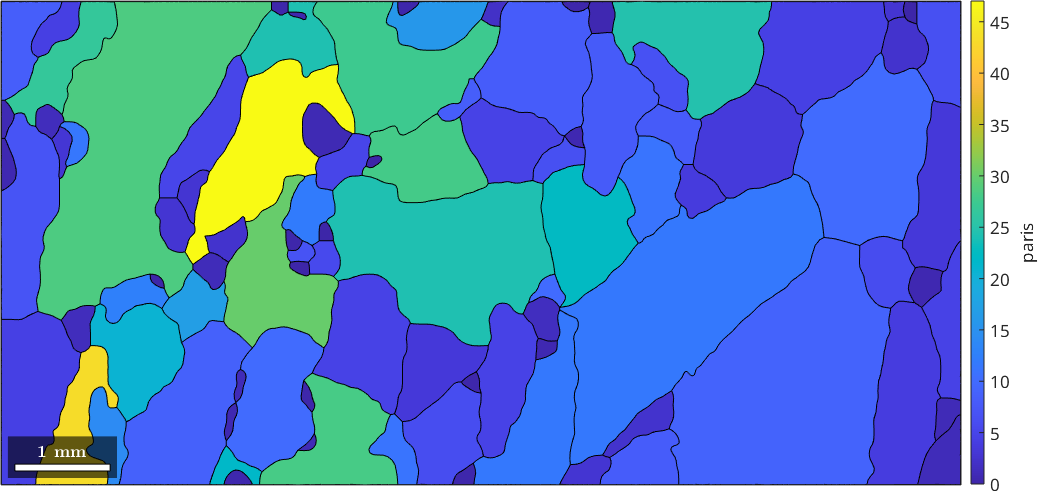## Fractal Dimension

The fractal dimension of grain boundaries has been used to characterize the conditions of dynamical recrystallization. One way to define the fractal dimension of grain boundaries is to look at the slope of the perimeter as a function of the equivalent radius of the grains. More precisely, we consider this relationship in a log log plot and fit a linear model.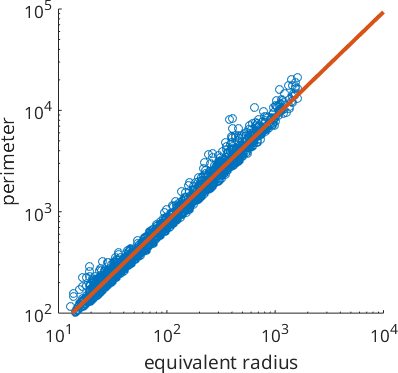The slope of the linear function is the fractal dimension.

It is important to understand that the fractal dimension computed this way heavily depends on the smoothing applied to the grain boundaries.